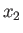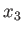Next: What is a Fluid? Up: Mathematical Models of Fluid Previous: Mathematical Models of Fluid

# Introduction

This chapter sets forth the mathematical models commonly used to describe the equilibrium and dynamics of fluids. Unless stated otherwise, all of the analysis is performed using a standard right-handed Cartesian coordinate system: (,,). Moreover, the Einstein summation convention is employed (which means that repeated roman subscripts are assumed to be summed from 1 to 3). (See Appendix B.) More information on the fundamental assumptions that underlie conventional fluid mechanics, as well as the basic equations that describe fluid motion, can be found in Batchelor 2000.

Richard Fitzpatrick 2016-03-31